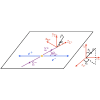Physical Review Letters ( IF 8.385 ) Pub Date : 2020-07-31 , DOI: 10.1103/physrevlett.125.052004
M. Ablikimet al.(BESIII Collaboration)From $1310.6×{10}^{6}\text{\hspace{0.17em}}\text{\hspace{0.17em}}J/\psi$ and $448.1×{10}^{6}\text{\hspace{0.17em}}\text{\hspace{0.17em}}\psi \left(3686\right)$ events collected with the BESIII experiment, we report the first observation of ${\mathrm{\Sigma }}^{+}$ and ${\overline{\mathrm{\Sigma }}}^{-}$ spin polarization in ${e}^{+}{e}^{-}\to J/\psi \left[\psi \left(3686\right)\right]\to {\mathrm{\Sigma }}^{+}{\overline{\mathrm{\Sigma }}}^{-}$ decays. The relative phases of the form factors $\mathrm{\Delta }\mathrm{\Phi }$ have been measured to be $\left(-15.5±0.7±0.5\right)°$ and $\left(21.7±4.0±0.8\right)°$ with $J/\psi$ and $\psi \left(3686\right)$ data, respectively. The nonzero value of $\mathrm{\Delta }\mathrm{\Phi }$ allows for a direct and simultaneous measurement of the decay asymmetry parameters of ${\mathrm{\Sigma }}^{+}\to p{\pi }^{0}\left({\alpha }_{0}=-0.998±0.037±0.009\right)$ and ${\overline{\mathrm{\Sigma }}}^{-}\to \overline{p}{\pi }^{0}\left({\overline{\alpha }}_{0}=0.990±0.037±0.011\right)$, the latter value being determined for the first time. The average decay asymmetry, $\left({\alpha }_{0}-{\overline{\alpha }}_{0}\right)/2$, is calculated to be $-0.994±0.004±0.002$. The $CP$ asymmetry ${A}_{CP,\mathrm{\Sigma }}=\left({\alpha }_{0}+{\overline{\alpha }}_{0}\right)/\left({\alpha }_{0}-{\overline{\alpha }}_{0}\right)=-0.004±0.037±0.010$ is extracted for the first time, and is found to be consistent with $CP$ conservation.

down
wechat
bug